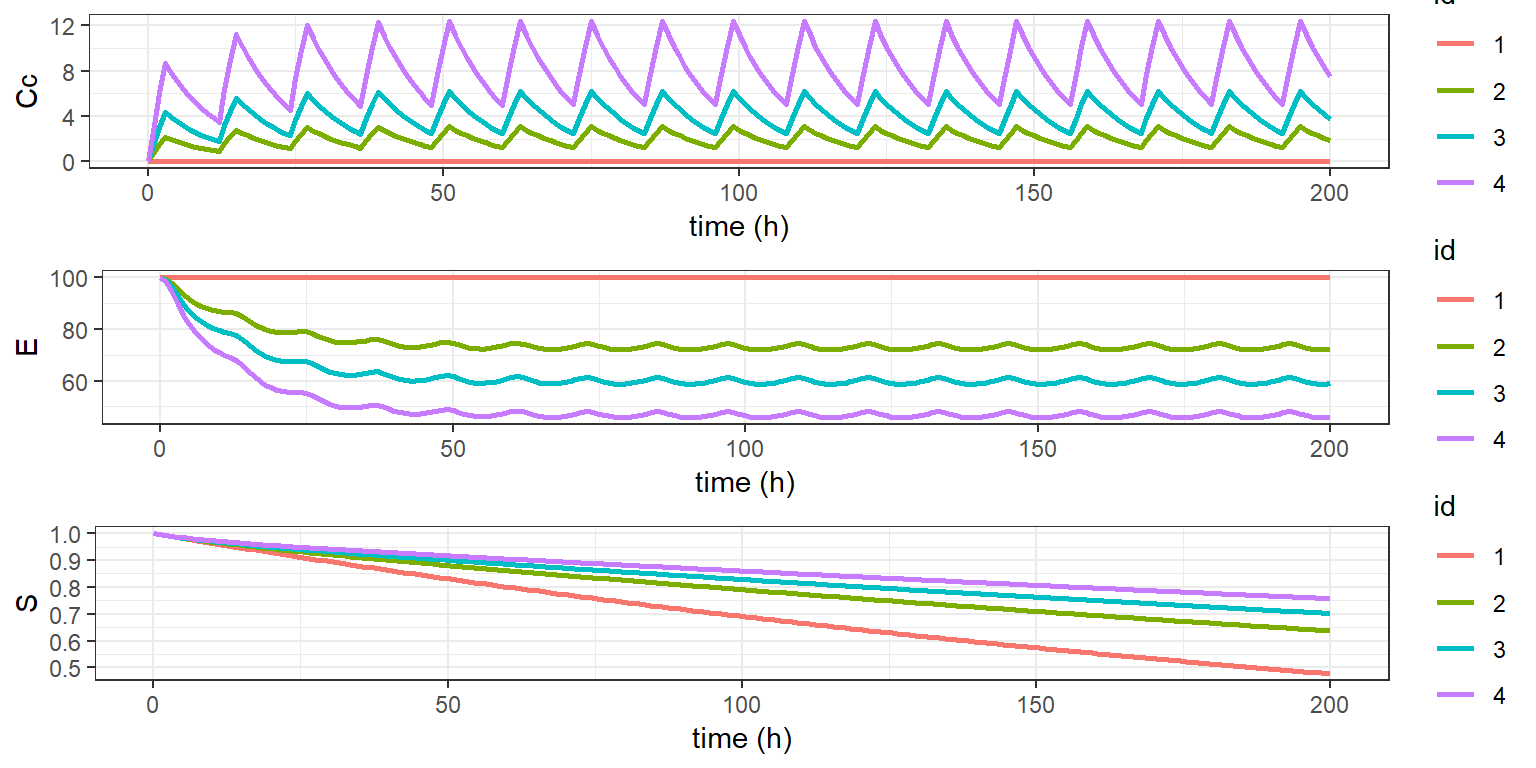The R codes and the Mlxtran model files can be found here

An optimal dose-regimen for the treatment of cancer is critical to maximise the effect while keeping the toxicity acceptable. PK/PD simulations are great way to explore all possible dose-regimen scenarios and to identify the one with the best efficacy-to-toxicity ratio. In this example we will focus on efficacy. We will compare and assess how four different dose-regimen will impact survival.

The example is structured in three parts. In the first part we simulate and compare the concentration, effect and survival for a single patient. The second part will include the between-patient variablity. The third part will investigate the full study design via Monte-Carlo clinical trial simulations and how the study size impacts our ability to retreive the drug effect on survival.

# The PKPD model

For this example we will work with a PK/PD model where the parameters have been previously estimated and are known. The PK model is a one compartment model for oral administration with zero order absorption and linear elimination.

The PD is modeled in two parts. The first part describes the underlying disease process via a turnover model and the second part links the disease process with survival. The drug effect is modeled as an inhibition of the turnover model input.

The objective of this first part is to compare the predicted concentration, effect and survival. There is no observation and no inter-individual variability for the moment.

The PKPD model is implemented in the text file model/cts2I.txt:

[LONGITUDINAL]
input={Tk0,V,Cl,Imax,E0,IC50,kout,alpha,beta}

EQUATION:
Cc = pkmodel(Tk0, V, Cl)

E_0 = E0
kin = E0*kout
ddt_E = kin*(1-Imax*Cc/(Cc+IC50)) - kout*E

h = (alpha/1000)*exp(beta*E)
H_0 = 0
ddt_H = h
S = exp(-H)


# Computing predictions

To run simulations with simulx the model parameter and the simulation design need to be specified in addtion to the structural model. Our study design has four treatment groups (defined as groups in simulx). Each group receive a dose every 12 hours, starting at time 0. For each of the group a different dose will be tested.

adm.time <- seq(0,200,by=12)
g1   <- list(treatment = trt1)
g2   <- list(treatment = trt2)
g3   <- list(treatment = trt3)
g4   <- list(treatment = trt4)

The same PKPD parameters are used for the four groups.

ppk  <- c(Tk0   = 3,   V    = 10,  Cl   = 1)
ppd  <- c(Imax  = 0.8, E0   = 100, IC50 = 4, kout = 0.1)
ptte <- c(alpha = 0.5, beta = 0.02)

We specify the output for simulx by defining a list output. Here, this list tells simulx to compute the predicted concentration $$Cc$$, the predicted effect $$E$$ and the predicted survival function $$S$$ at every hour, starting at time 0:

f <- list(name = c('Cc','E','S'),
time = seq(0,200,by=1))

We can now run simulx with these input arguments

res <- simulx(model     = "model/cts2I.txt",
group     = list(g1,g2,g3,g4),
parameter = list(ppk, ppd, ptte),
output    = f)
names(res)
##  "Cc"        "E"         "S"         "treatment"

and plot the results

library(gridExtra)

plot1 <- ggplot(data=res$Cc, aes(x=time, y=Cc, colour=id)) + geom_line(size=1) + xlab("time (h)") + ylab("Cc") plot2 <- ggplot(data=res$E,  aes(x=time, y=E,  colour=id)) +
geom_line(size=1) + xlab("time (h)") + ylab("E")
plot3 <- ggplot(data=res\$S,  aes(x=time, y=S,  colour=id)) +
geom_line(size=1) + xlab("time (h)") + ylab("S")
grid.arrange(plot1, plot2, plot3, ncol=1)These simulation are for a single individual. To have more realisting predictions we will run simulation of a population PKPD model. We will see in part II how to compare these same quantities, but taking into account the inter-individual variability of the PKPD parameters.

part I     part II     part III